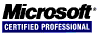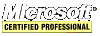# JavaScript Tutorial

#### Date/Time Component Methods

`getTime()` returns the milliseconds representation of the date; same as `valueOf()`.
`setTime(milliseconds)` sets the milliseconds representation of the date, thus changing the entire date.
`getFullYear()` returns the four-digit year (2007 instead of just 07).
`getUTCFullYear()` returns the four-digit year of the UTC date value.
`setFullYear(year)` sets the year of the date. The year must be given with four digits (2007 instead of just 07).
`setUTCFullYear(year)` sets the year of the UTC date. The year must be given with four digits (2007 instead of just 07).
`getMonth()` returns the month of the date, where 0 represents January and 11 represents December.
`getUTCMonth()` returns the month of the UTC date, where 0 represents January and 11 represents December.
`setMonth(month)` sets the month of the date, which is any number 0 or greater. Numbers greater than 11 add years.
`setUTCMonth(month)` sets the month of the UTC date, which is any number 0 or greater. Numbers greater than 11 add years.
`getDate()` returns the day of the month (1 through 31) for the date.
`getUTCDate()` returns the day of the month (1 through 31) for the UTC date.
`setDate(date)` sets the day of the month for the date. If the date is greater than the number of days in the month, the month value also gets increased.
`setUTCDate(date)` sets the day of the month for the UTC date. If the date is greater than the number of days in the month, the month value also gets increased.
`getDay()` returns the date's day of the week as a number (where 0 represents Sunday and 6 represents Saturday).
`getUTCDay()` returns the UTC date's day of the week as a number (where 0 represents Sunday and 6 represents Saturday).
`getHours()` returns the date's hours as a number between 0 and 23.
`getUTCHours()` returns the UTC date's hours as a number between 0 and 23.
`setHours(hours)` sets the date's hours. Setting the hours to a number greater than 23 also increments the day of the month.
`setUTCHours(hours)` sets the UTC date's hours. Setting the hours to a number greater than 23 also increments the day of the month.
`getMinutes()` returns the date's minutes as a number between 0 and 59.
`getUTCMinutes()` returns the UTC date's minutes as a number between 0 and 59.
`setMinutes(minutes)` sets the date's minutes. Setting the minutes to a number greater than 59 also increments the hour.
`setUTCMinutes(minutes)` sets the UTC date's minutes. Setting the minutes to a number greater than 59 also increments the hour.
`getSeconds()` returns the date's seconds as a number between 0 and 59.
`getUTCSeconds()` returns the UTC date's seconds as a number between 0 and 59.
`setSeconds(seconds)` sets the date's seconds. Setting the seconds to a number greater than 59 also increments the minutes.
`setUTCSeconds(seconds)` sets the UTC date's seconds. Setting the seconds to a number greater than 59 also increments the minutes.
`getMilliseconds()` returns the date's milliseconds.
`getUTCMilliseconds()` returns the UTC date's milliseconds.
`setMilliseconds(milliseconds)` sets the date's milliseconds.
`setUTCMilliseconds(milliseconds)` sets the UTC date's milliseconds.
`getTimezoneOffset()` returns the number of minutes that the local time zone is off set from UTC. For example, Eastern Standard Time returns 300. This value changes when an area goes into Daylight Saving Time.

## The Browser Object Model

### The Window Object

The `window` object is the core of the Browser Object Model.

#### Frames

A page with frames has one window object per frame.

``````<html>
<title>Frameset Page</title>
<frameset rows="100,*">
<frame src="top_frame.htm" name="top_frame">
<frameset cols="50%,50%">
<frame src="left_frame.htm" name="left_frame">
<frame src="right_frame.htm" name="right_frame">
</frameset>
</frameset>
</html>
``````

This diagram demonstrates how to access the frames.

 ``````window.frames; window.frames["top_frame"]; top.frames; top.frames["top_frame"]; frames; frames["top_frame"]; `````` ``````window.frames; window.frames["left_frame"]; top.frames; top.frames["left_frame"]; frames; frames["left_frame"]; `````` ``````window.frames; window.frames["right_frame"]; top.frames; top.frames["right_frame"]; frames; frames["right_frame"]; ``````

The `parent` object points to the current frame's immediate parent frame. Parent may be equal to top and when there are no frames then parent is equal to top and both are equal to window.

<< < [Page 12 of 22] > >>